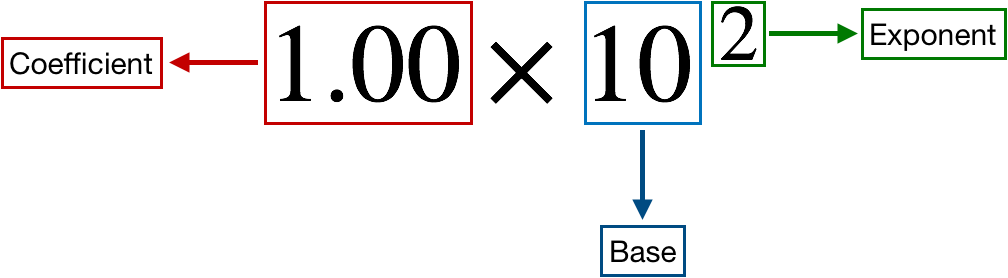# Problem: The circumference of Earth through the poles is 40,008 km. Round this number to four significant figures, and express it in standard exponential notation.

###### FREE Expert Solution

We're asked to round off the circumference of Earth which is 40,008 km to four significant figures and to express it in standard exponential notation

Recall that a scientific notation expression is composed of three parts:89% (354 ratings)###### Problem Details

The circumference of Earth through the poles is 40,008 km. Round this number to four significant figures, and express it in standard exponential notation.